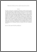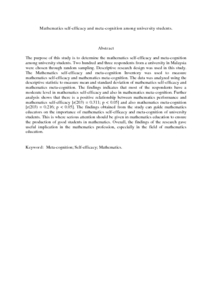# Mathematics self-efficacy and meta-cognition among university students

## Citation

Wan Jaafar, Wan Marzuki and Mohd Ayub, Ahmad Fauzi (2010) Mathematics self-efficacy and meta-cognition among university students. Procedia - Social and Behavioral Sciences, 8. pp. 519-524. ISSN 1877-0428

## Abstract

The purpose of this study is to determine the mathematics self-efficacy and meta-cognition among university students. Two hundred and three respondents from a university in Malaysia were chosen through random sampling. Descriptive research design was used in this study. The Mathematics self-efficacy and meta-cognition Inventory was used to measure mathematics self-efficacy and mathematics meta-cognition. The data was analyzed using the descriptive statistic to measure mean and standard deviation of mathematics self-efficacy and mathematics meta-cognition. The findings indicates that most of the respondents have a moderate level in mathematics self-efficacy and also in mathematics meta-cognition. Further analysis shows that there is a positive relationship between mathematics performance and mathematics self-efficacy [r(203) = 0.311; p < 0.05] and also mathematics meta-cognition [r(203) = 0.216; p < 0.05]. The findings obtained from the study can guide mathematics educators on the importance of mathematics self-efficacy and meta-cognition of university students. This is where serious attention should be given in mathematics education to ensure the production of good students in mathematics. Overall, the findings of the research gave useful implication in the mathematics profession, especially in the field of mathematics education.Preview
PDF (Abstract)
Mathematics selfefficacy and meta-cognition among university students..pdfView Item